# Algorithms

### What is it?

An algorithm is a series of ordered steps that are required to solve a problem. In order to work, algorithms need to have their steps in the right order.

Algorithms are everywhere in our daily lives. At their simplest level, algorithms exist as a series of instructions that enable us to solve simple problems; for example, recipes or getting ready for the day. Algorithms can also be more complex. For example, some of the largest corporations use algorithms to guide our internet browsing, airports use them to program flight paths, and the health sector uses them to find donor–patient matches.

#### Australian Curriculum definition

##### Algorithms

Step-by-step procedures required to solve a problem. For example, to find the largest number in a list of positive numbers:

• Note the first number as the largest.
• Look through the remaining numbers, in turn, and if a number is larger than the number found in 1, note it as the largest.
• Repeat this process until complete. The last noted number is the largest in the list.

An algorithm may be described in many ways. Flowcharts are often useful in visualising an algorithm.What are algorithms?

This 5-minute video explores the making of a Vegemite sandwich and other processes to explain algorithms.Problem-solving using algorithms

This 6-minute video explores some of the criteria and complexities of creating an algorithm that solves problems efficiently.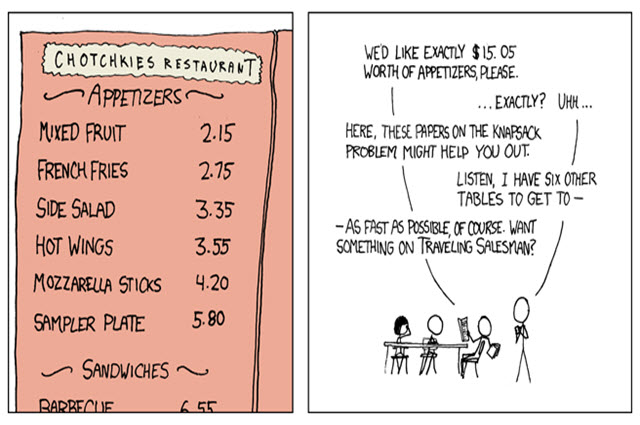CS Unplugged: Field guide: Complexity and tractability

This chapter covers problems where it's easy to tell the computer what to do – by writing a program – but the computer can't do what we want because it takes far too long. Find out about what is meant by tractable and intractable problems. Learn about complexity, why it is an important concept and how it relates to algorithms.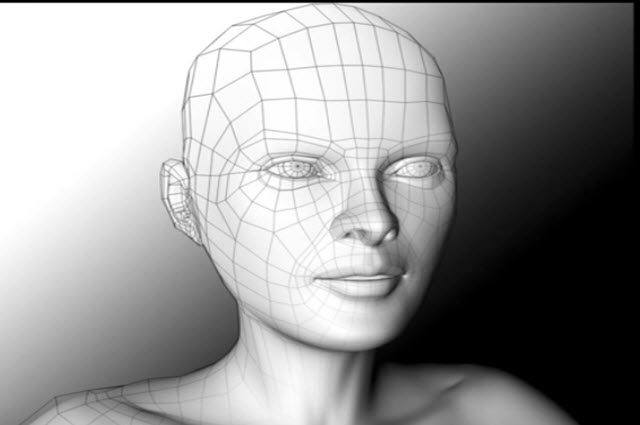Intro to algorithms

Use this website to develop your understanding of algorithms, including searching, sorting, recursion and graph theory. Learn with a combination of articles, visualisations, quizzes and coding challenges.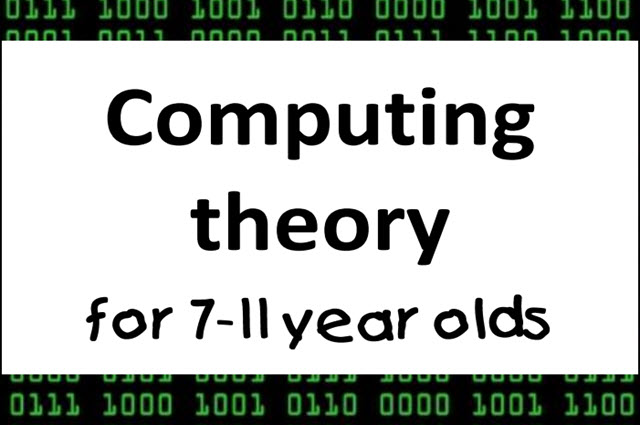Computing theory for 7–11 year olds

This resource provides information for teachers about various topics including algorithms.CS Unplugged: Field guide: Algorithms

This chapter covers what an algorithm is, and why they are such a fundamental idea in computer science. Find out more about the two most common and important types of algorithms – searching and sorting.Intro to Algorithms: Crash Course Computer Science #13

See how algorithms help us complete tasks more efficiently. Learn about sorting algorithms in this Crash Course video.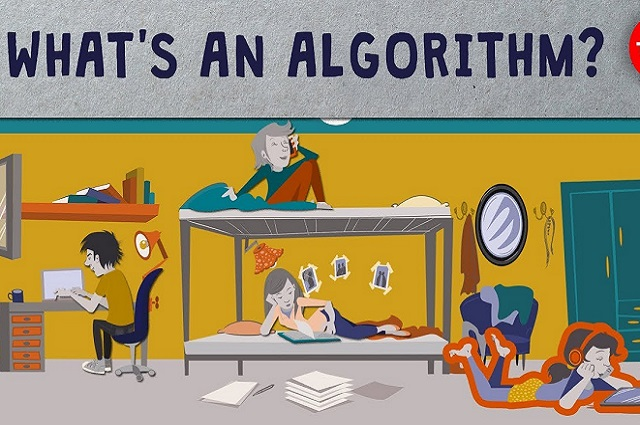What's an algorithm? - David J Malan

Learn about pseudocode using a problem of counting people in a room.

#### How to teach it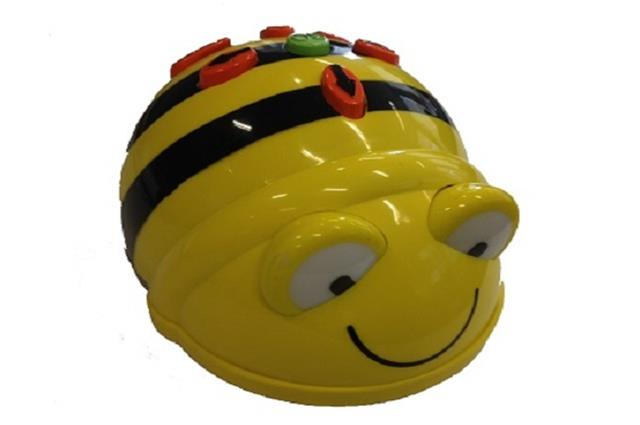Buzzing with Bee-Bots

Students follow and describe a series of steps to program a floor robot. Plan a route to program a robot to follow a path and write a sequence of steps (algorithm).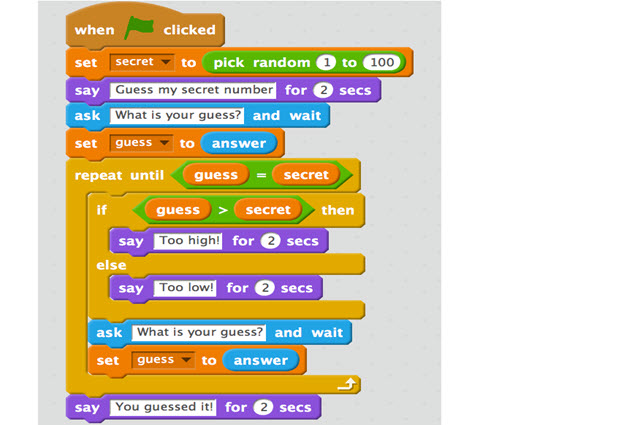Getting warmer

This lesson sequence intentionally uses a visual based programming tool to introduce designing and validating algorithms. Those students who complete this task can move to code the result in any text-based language with which they are familiar.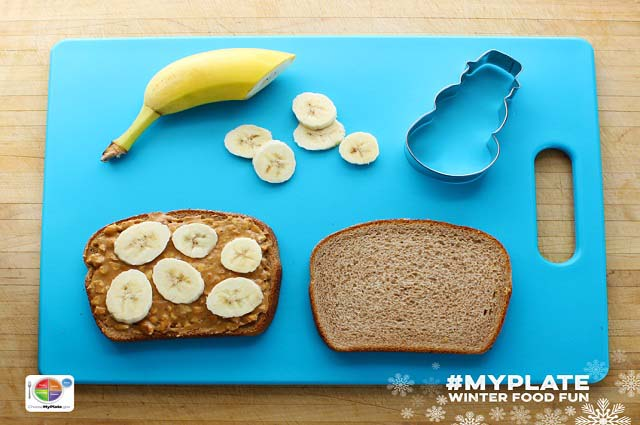Introducing algorithms

Students design a sequence of steps for others to follow. They convey their instructions to peers and evaluate the work of others to determine if the outcome was successful.Visual to text coding lesson 1: Temperature converter

Use this resource to introduce and reinforce students understanding of algorithms represented diagrammatically and using pseudocode.Take a LEGO® building challenge

In pairs, explore giving and following a sequence of steps and decisions to build a LEGO® toy.There can only be one

Students write a simple suite of programs that can be used to facilitate an SRC election though the collection and processing of data. It assumes that students have been introduced to Python programming language.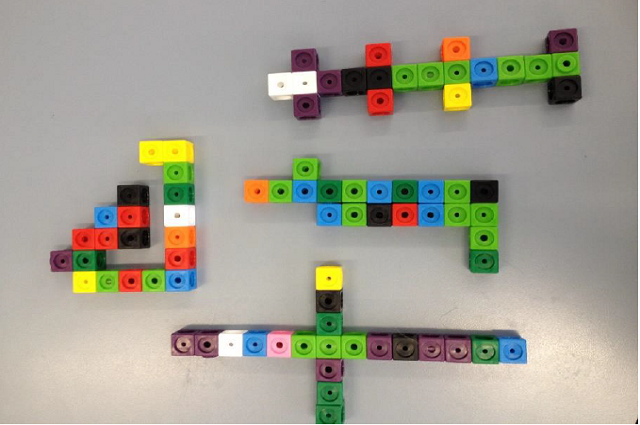Snap block models

Create a model using snap blocks one-block high and create a code so someone else can build your model.Breaking up can be good

This sequence provides a gentle introduction to the skill of decomposition by having students develop discrete modules that together serve a single need: a maths teacher asks for a program that can be used to demonstrate aspects of maths. This sequence can be used in conjunction with 'Comparing and selecting appropriate algorithms'.Understanding algorithms and the smiley face biscuit challenge

Algorithms are a key concept in Digital Technologies. They help us follow, describe and represent a sequence of steps and decisions needed to solve problems. This resource explores some idea for following algorithms including the smiley face biscuit visual algorithm challenge.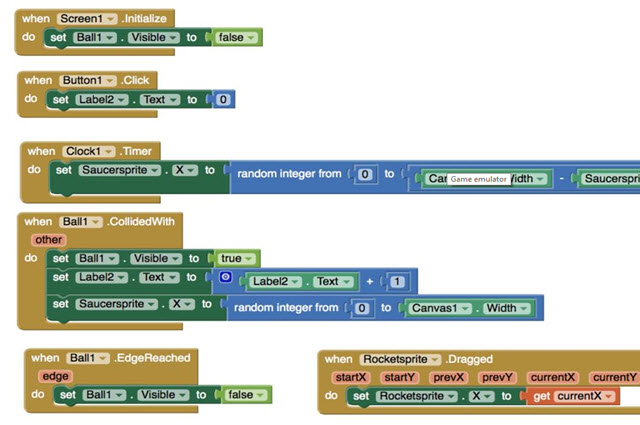Behaving with real class

One challenge in teaching object-oriented principles is finding a suitable programming language. Many of these languages are too complex and their environments too confusing. This lesson sequence offers a choice of one of two approaches in an attempt to address this problem.Water water everywhere!

In this lesson, students are presented with the challenging problem of measuring a volume of water using containers that are not the exact measurement size. Students will decompose a complex problem into discrete steps, design an algorithm for solving the problem, and evaluate solution efficiencies and optimisation in a simulation.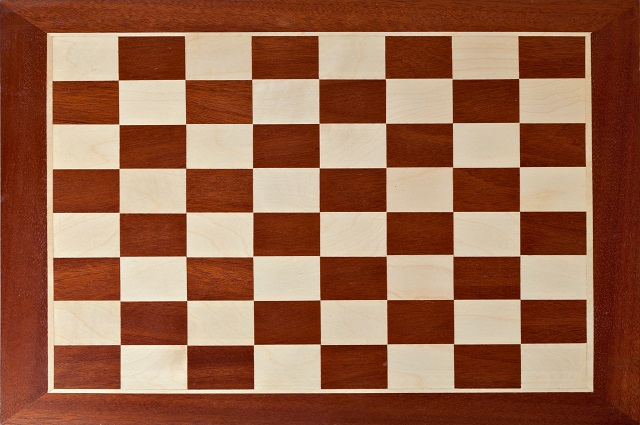Who wants to be a millionaire?

Drawing on the well-known wheat/rice and chessboard problem, students use spreadsheets to simulate iteration and to solve problems.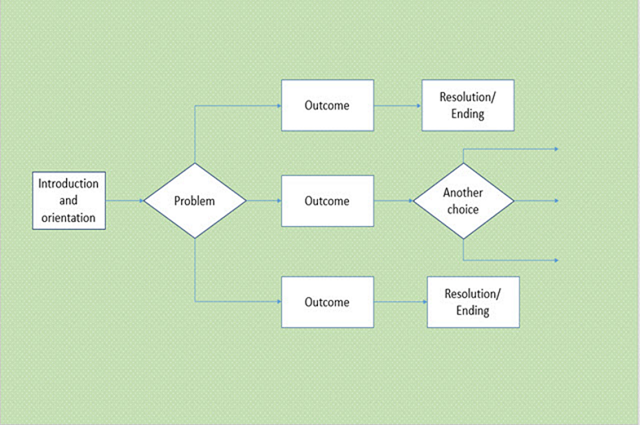Students create a storyboard to plan a ‘choose your own adventure' story, where the reader is provided with a number of decisions that lead to alternative endings.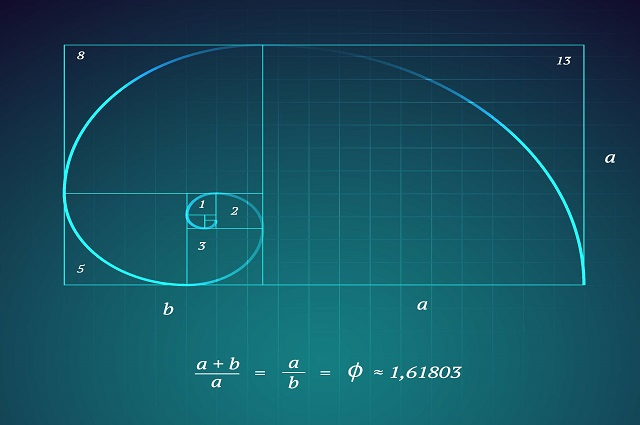Fibonacci served three ways

Students learn to code separate modules that perform discrete functions but collectively meet the needs of the solution. They select the most appropriate algorithm based on the type of problem.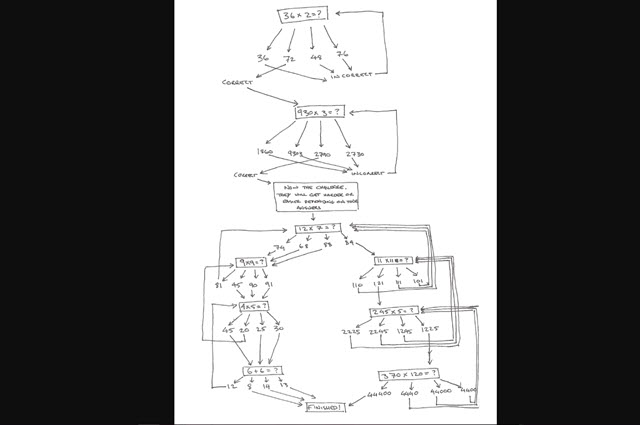Making maths quizzes 1: plan and test our programs

In this sequence students plan, create and edit a program that asks maths questions that are harder or easier depending on user performance.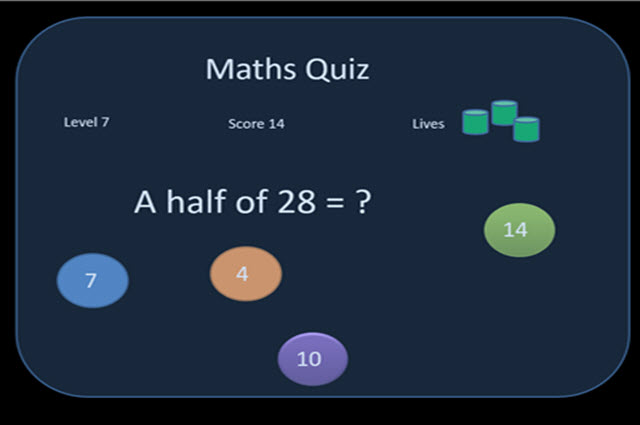Making maths quizzes 2: implementing a digital solution

In this sequence students implement a digital solution for a maths quiz. They test and assess how well it works.Planting fruit and vegetables

This sequence integrates science as students grow a plant from seed, capture each step and decision as an algorithmic process and record data for future learning.Eco-calculator

Students make a paper prototype of an eco-calculator to demonstrate human impact on the environment and suggest changes in behaviour. This is an unplugged learning sequence with opportunities to extend learning through the development of a Scratch quiz.

#### For the classroom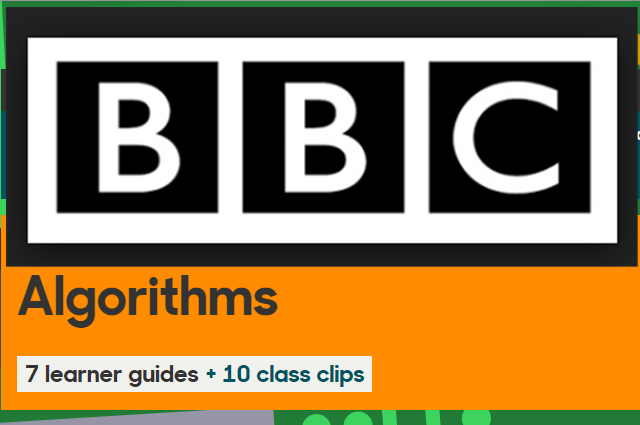BBC computer science algorithms

This is a collection of learner guides and videos about algorithms.A guessing game

This game will give you an idea of how different algorithms for the same problem can have wildly different efficiencies.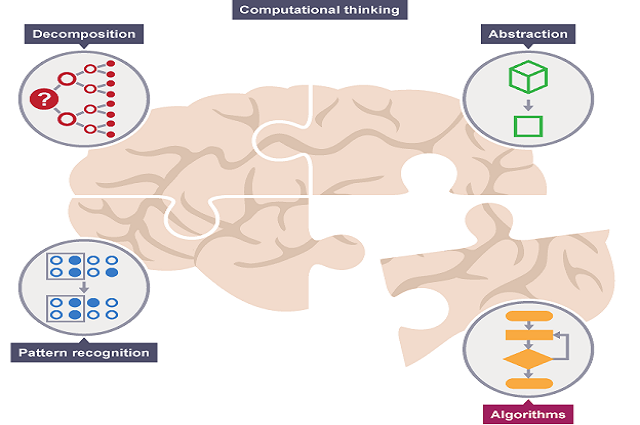BBC bitesize: what is an algorithm?

Use this resource to introduce algorithms.

#### What other schools are doing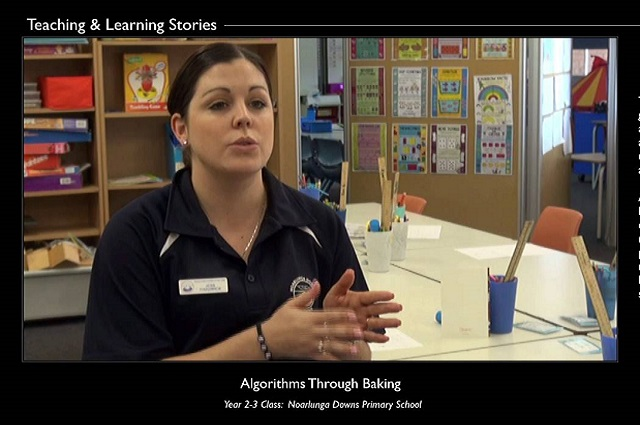Noarlunga Downs Primary School: Algorithms through baking (part 1)

Understanding algorithms through baking.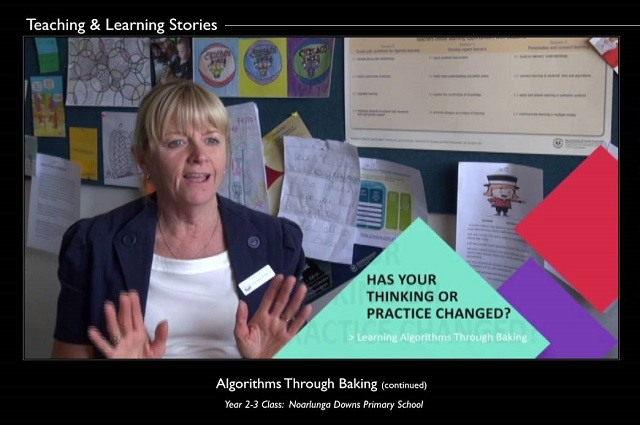Noarlunga Downs Primary School: Algorithms through baking (part 2)

Understanding algorithms through baking.

#### Levels F - 2

Follow, describe and represent a sequence of steps and decisions (algorithms) needed to solve simple problems (ACTDIP004)

#### Levels 3 - 4

Define simple problems, and describe and follow a sequence of steps and decisions (algorithms) needed to solve them (ACTDIP010)

Implement simple digital solutions as visual programs with algorithms involving branching (decisions) and user input (ACTDIP011)

#### Levels 5 - 6

Design, modify and follow simple algorithms involving sequences of steps, branching, and iteration (repetition) (ACTDIP019)

#### Levels 7 - 8

Design algorithms represented diagrammatically and in English, and trace algorithms to predict (ACTDIP029)

#### Levels 9 - 10

Design algorithms represented diagrammatically and in structured English and validate algorithms and programs through tracing and test cases (ACTDIP040)

Implement modular programs, applying selected algorithms and data structures including using an object-oriented programming language (ACTDIP041)